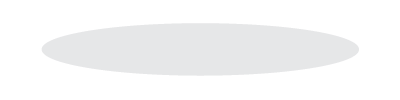# Chapter 10: Perfect Competition

1. Overview
1. Basic Concepts
1. Demand Curve of Market/Industry
1. Downward sloping to the right
2. Demand Curve of the Individual Firm
1. Is horizontal
1. implies a perfectly elastic demand function
2. therefore: P = MR for the firm
3. Firms are price-takers
1. the price remains constant
2. the only decision: the optimal quantity to produce given a price
4. P = MR
1. implies that there is no pricing strategy
5. P = MC
1. implies that the price of the product is equal to the extra cost incurred by the seller in producing the marginal or extra unit of production
6. P = Minimum Average Cost
1. implies that firms are operating at zero economic profits
2. efficiency has been attained
1. each increase in the cost of an extra unit of production equals the price that the consumer is willing to pay
7. ​​Short run
1. price as reflected by the demand function remains horizontal but can increase or decrease in the short run
2. there can be profits or losses
3. the firm want to maximize profits by producing the optimal output (the level at which MR = MC)
1. in perfect competition, MR = P
8. ​​At long-run equilibrium:
1. P = MR
2. P = MC
3. P = Minimum average cost
4. firms realize zero economic profit or normal profits
5. firms have no incentive to price their goods and services below the market price
6. the demand function for each firm is horizontal
7. products are homogenous
8. therefore: no need for advertising
9. Profits and competition
1. normal profits
1. zero economic profits
2. economic losses
1. profits less than normal
3. economic profits
1. greater than normal profits
10. ​​For each price (P = MR) in the short run:
1. If P < AVC, firm should shut down
1. total losses = total fixed cost (FTC)
2. If P > AVC, firm continues to produce as long as MR > MC up to the level of output at which profits are maximized (at which  MR = MC)
11. Price elasticity of supply
1. greater in the long run than in the short run since there are more opportunities for substitution of inputs
2. ​​​The Expanded Concepts
1. The Individual Firm as a Price-Taker
1. Reasons firms must sell at the market price:
1. if they increase the price, all customers will buy the identical product from another seller
2. if they lower the price, it will incur losses and not increase sales significantly
1. because each firm only has a very small share of the market
2. ​​​Strategies for Profit-Maximizing in the Short-Run
1. If the firm can’t cover average variable costs with its price, it is more economical to shut down production and simply incur fixed costs
1. fixed costs- don’t vary with output changes
2. The firm should produce if P > AVC (average variable costs)
1. should continue to as long as MR > MC
1. means that the firm is adding more to its revenue than it is adding to its costs at the margin of producing one additional unit
2. MR – marginal revenue
3. MC – marginal cost
3. ​​Optimal level of output
1. occurs at MR = MC
1. if impossible, then at the highest level of output at which MR > MC
4. ​​To determine the total profits or total losses at the optimal level of output:
1. total profits = Q(P – ATC)
2. Q – optimal level of output
3. ​​Decision Making for Three Situations
1. Shut-down case
1. optimal output is zero
2. ​P < AVC, so firm should shut down (at least temporarily)
2. Profit maximization case
1. P > AVC, so firm should continue to produce as long as MR > MC up to the level of output at which MR = MC (optimal)
3. Loss minimization
1. P > AVC, so the firm will continue to produce as long as MR > MC up the level of output at which MR =MC even if there are losses
2. MR = MC minimizes total losses
4. ​​Evaluation of Perfect Competition
1. In the short run:
1. the supply curve is identified as that portion of the MC (marginal cost) curve above its intersection with the AVC (average variable cost) curve
1. the firm will only produce if the market price is greater than their average variable costs
2. ​​Efficiency
1. consumers buy what they want at prices they pay that are equal to the minimum average cost and the marginal cost of production
2. producers gain from the difference between the price they are willing to supply goods and the price they receive in the market
3. ​​​No “rent-seeking”
1. firms cannot extract from the market any more than they contribute to the market at the margin of any transaction
2. zero economic profits in the long run
5. ​​The Long Run
1. Assumptions
1. the only adjustment made, as demand changes, is that new firms enter (demand increases leading to short run profits)
1. or: the present firms increase production
2. the firms’ average cost curves are identical and these are unaffected by the adjustment process
3. new firms enter with the same cost curves as the present firms
1. the new firms are attracted by the short run profits in the industry
4. firms will exit from the industry when demand decreases, leading to short term losses
2. Efficiency is produced in the long run of perfect competitionYou just finished Chapter 10: Perfect Competition. Nice work!

Tip: Use ← → keys to navigate!

### How to cite this note (MLA)

Aboukhadijeh, Feross. "Chapter 10: Perfect Competition" StudyNotes.org. Study Notes, LLC., 12 Oct. 2013. Web. 03 Oct. 2023. <https://www.apstudynotes.org/microeconomics/outlines/chapter-10-perfect-competition/>.